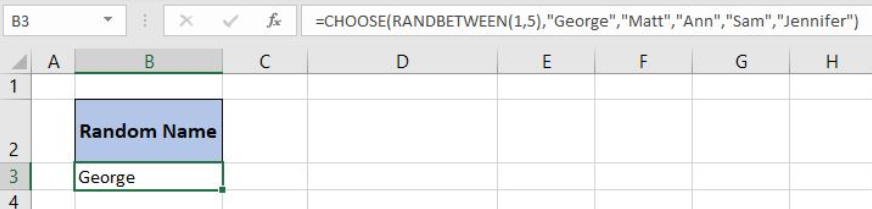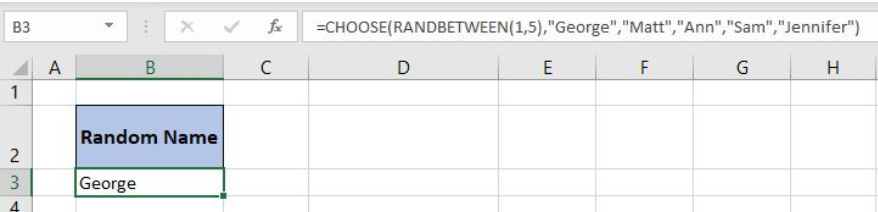Get instant live expert help with Excel or Google Sheets“My Excelchat expert helped me in less than 20 minutes, saving me what would have been 5 hours of work!”

#### Post your problem and you’ll get expert help in seconds.

Your message must be at least 40 characters
Our professional experts are available now. Your privacy is guaranteed.

# Find Out How to Generate Random Names in Excel

Excel allows us to get a random number from a range using the CHOOSE and  RANDBETWEEN functions. This step by step tutorial will assist all levels of Excel users to learn how to randomly generate words in Excel.Figure 1. The final result of the formula

## Syntax of the RANDBETWEEN Formula

The generic formula for the RANDBETWEEN function is:

`=RANDBETWEEN(bottom, top)`

The parameters of the RANDBETWEEN function are:

• bottom – a value from which we want to get a random value
• top – a value to which we want to get a random value.

## Syntax of the CHOOSE Formula

The generic formula for the CHOOSE function is:

`=CHOOSE(index_num, value1, [value2], [value3],...)`

The parameters of the CHOOSE function are:

• index_num – an index of the value which we want to retrieve
• value1, [value2], [value3],… – a list of values from which one value is returned.

## Get a Random Name Using the Formula

In the cell B3, we want to get a random name from the name list: “Mike”, “John”, “Lilly”, “Robert” and “Jennifer”.

The formula looks like:

`=CHOOSE(RANDBETWEEN(1,5), "George", "Matt", "Ann", "Sam", "Jennifer")`

The parameter bottom of the RANDBETWEEN function is 1 and the top is 5. The result of this function is a random number between 1 and 5 which is the index_num parameter of the CHOOSE function. The values of the function are “George”, “Matt”, “Ann”, “Sam”, “Jennifer”.

To apply the formula, we need to follow these steps:

• Select cell B3 and click on it
• Insert the formula: `=CHOOSE(RANDBETWEEN(1,5),"George","Matt","Ann","Sam","Jennifer")`
• Press enter.Figure 2. Using the formula to get a random name from the range

If we evaluate the function, we can see that the RANDBETWEEN function returns number 1. Therefore, the CHOOSE function returns the first value from the values. Finally, the result in the B3 cell is “George”.

Most of the time, the problem you will need to solve will be more complex than a simple application of a formula or function. If you want to save hours of research and frustration, try our live Excelchat service! Our Excel Experts are available 24/7 to answer any Excel question you may have. We guarantee a connection within 30 seconds and a customized solution within 20 minutes.

Solution examplesI'm Apply a =RANDBETWEEN formula in one cells want to apply Formula to entire column and run formula when i hit the entire on the particular cell
Solved by M. S. in 20 minsHelp! In a coin toss I have a 1 in 2 (fifty-fifty) chance of getting heads or tails. I need a formula to determine my chance of getting 4 heads in a row or 4 tails in a row out of 10 coin tosses. If I get 4 in a row I win. What formula can I use to determine my chances of winning if I repeat the 10 tosses for hundreds or thousands of times? I get as far as =RANDBETWEEN(0,1) but I have not been able to figure out what formula to use to determine if there are 4 in a row in a group of 10 tosses, times thousands of groups with 10 tosses in each group. Thank-you for any help you can provide.
Solved by V. F. in 51 minsI have 4 column. when i fill the first 3 column. in 4th column i need a unique id number and i generate unique id number using RANDBETWEEN formula [ =CHAR(RANDBETWEEN(65,90))&CHAR(RANDBETWEEN(65,90))&RANDBETWEEN(1000,9900) ] but i want ..... when i'm filling the 3 column after that 4th column run this formula and generate unique id automatically
Solved by X. Q. in 20 minswhen i fill column A and B then C column generate Unique ID using RandBetween Formula {=CHAR(RANDBETWEEN(65,90))&CHAR(RANDBETWEEN(65,90))&RANDBETWEEN(1000,9900)} ...
Solved by S. Q. in 20 mins